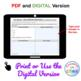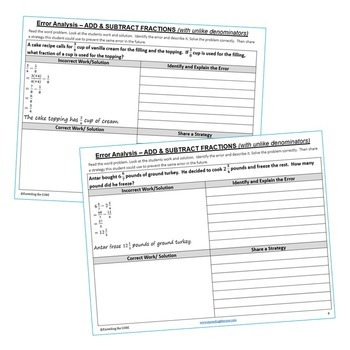# ADD & SUBTRACT FRACTIONS (UNLIKE DENOMINATORS) Error Analysis - Find the ErrorSubject
Resource Type
File Type
PDF (1 MB|14 pages)
Standards
\$3.00
Also included in:
1. ADD & SUBTRACT FRACTIONS BUNDLE - Graphic Organizers, Error Analysis, Maze, Riddle, Coloring Activity This BUNDLE includes 10 error analysis activities, 10 problem solving graphic organizers, 1 maze, 1 riddle, 1 coloring activity (over 45 skills practice and real-world word problems). The resou
\$8.00
\$6.40
Save \$1.60
2. Have your students apply their understanding of 5th grade math concepts with these ERROR ANALYSIS activities. This GROWING BUNDLE resource includes 6 sets (a total of 60 questions) of Common Core WORD PROBLEMS that are solved incorrectly. Students have to identify the error, provide the correct s
\$18.00
\$14.40
Save \$3.60
3. 5th Grade Math COMMON CORE Assessments, Warm-Ups, Error Analysis, Problem Solving Graphic Organizers, Task Cards, Mazes, Riddles, Coloring ActivitiesThis is a GROWING BUNDLE of all of the 5th Grade MATH RESOURCES currently in my store { 42 Resources / OVER 350 PAGES } PLUS ALL FUTURE 5th GRADE RES
\$126.50
\$88.55
Save \$37.95
• Product Description
• Standards
Have your students apply their understanding of ADDING & SUBTRACTING FRACTIONS (UNLIKE DENOMINATORS) with these ERROR ANALYSIS activities.

Benefits of Math Error Analysis:
Giving students opportunities to identify and correct errors in presented solutions allows them to show their understanding of the mathematical concepts you have taught.

Whats Included:
This resource includes 10 real-world word problem that are solved incorrectly. Students have to IDENTIFY THE ERROR, provide the CORRECT SOLUTION and share a helpful STRATEGY for solving the problem. An ANSWER KEY has also been provided with examples of possible answers. Be sure to download the sample for a full overview of what you get.

How to Use:
When I present this activity to my students, I tell them that these are errors that students from my other class made and I need their help to correct them. Students LOVE correcting other students mistakes. Students can use these activities with a partner, as a warm-up , as classwork, homework, in math centers or group work.

Be sure to download the sample for a full overview of what you get.

Topics Covered:
✔ Add and subtract fractions with unlike denominators.
✔ Add and subtract mixed numbers with unlike denominators.
✔ Rename to find the difference of two mixed numbers.

Common Core Alignment :
* 5.NF.1 - Add and subtract fractions with unlike denominators (including mixed numbers) by replacing given fractions with equivalent fractions in such a way as to produce an equivalent sum or difference of fractions with like denominators.
* MP3 - Construct viable arguments and critique the reasoning of others.
___________________________________________________________________________
This resource is included in the following bundles:
* 5th Grade Math MEGA BUNDLE
* Add & Subtract Fractions UNIT RESOURCE BUNDLE

More Fractions review:
* ADD & SUBTRACT FRACTIONS with UNLIKE DENOMINATORS Maze, Riddle, Coloring Page
* MULTIPLY AND DIVIDE FRACTIONS Error Analysis
* MULTIPLY AND DIVIDE FRACTIONS Task Cards

* 5th Grade Math Daily/Weekly REVIEW

If you would like to get updates on NEW and CURRENT resources...
FOLLOW the Exceeding the Core BLOG!
FOLLOW me on Teachers Pay Teachers!

© Exceeding the CORE. All rights reserved. Please note - this resource is for use by one teacher only. Additional teachers must purchase their own license. Copying, editing, selling, redistributing, or posting any part of this product on the internet is strictly forbidden. Violations are subject to the penalties of the Digital Millennium Copyright Act.
Construct viable arguments and critique the reasoning of others. Mathematically proficient students understand and use stated assumptions, definitions, and previously established results in constructing arguments. They make conjectures and build a logical progression of statements to explore the truth of their conjectures. They are able to analyze situations by breaking them into cases, and can recognize and use counterexamples. They justify their conclusions, communicate them to others, and respond to the arguments of others. They reason inductively about data, making plausible arguments that take into account the context from which the data arose. Mathematically proficient students are also able to compare the effectiveness of two plausible arguments, distinguish correct logic or reasoning from that which is flawed, and-if there is a flaw in an argument-explain what it is. Elementary students can construct arguments using concrete referents such as objects, drawings, diagrams, and actions. Such arguments can make sense and be correct, even though they are not generalized or made formal until later grades. Later, students learn to determine domains to which an argument applies. Students at all grades can listen or read the arguments of others, decide whether they make sense, and ask useful questions to clarify or improve the arguments.
Add and subtract fractions with unlike denominators (including mixed numbers) by replacing given fractions with equivalent fractions in such a way as to produce an equivalent sum or difference of fractions with like denominators. For example, 2/3 + 5/4 = 8/12 + 15/12 = 23/12. (In general, 𝘢/𝘣 + 𝘤/𝘥 = (𝘢𝘥 + 𝘣𝘤)/𝘣𝘥.)
Understand a fraction 𝘢/𝘣 with 𝘢 > 1 as a sum of fractions 1/𝘣.
Total Pages
14 pages